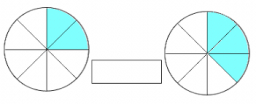# 1/12 fraction

Which statement about determining the quotient 1/12÷3 is true?

A.Because 1/36×3=1/12, 1/12 divided by 3 is 1/36.

B.Because 1/4×3=1/12, 1/12 divided by 3 is 1/4.

C.Because 3/4×3=1/12, 1/12 divided by 3 is 3/4.

D.Because 4/3×3=1/12, 1/12 divided by 3 is 4/3.

Result

x = (Correct answer is: A)### Step-by-step explanation:Did you find an error or inaccuracy? Feel free to write us. Thank you!

Tips for related online calculators
Need help calculating sum, simplifying, or multiplying fractions? Try our fraction calculator.